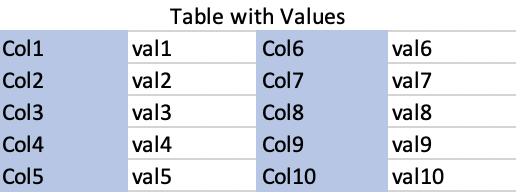# Table Flipped and In Two Parts

I have a table with one row which I am trying to flip (from being horizontal to vertical) and then shown with the columns and values being paired in two columns. I am not sure how to do either of these though. I added a screen capture of a table in excel to show what I hope the end product would look like.

Below is what I want my table to look like:This is what my code produces:

Below is my code:

``````from bokeh.io import show
from bokeh.models.widgets import DataTable, DateFormatter, TableColumn
import pandas as pd

df_dict = {}
for i in range(10):
col = 'col:' + str(i)
val = 'val:'+ str(i)
df_dict.update({col:val})

df = pd.DataFrame([df_dict])

def create_table(df):
Columns = [TableColumn(field=Ci, title=Ci) for Ci in df.columns] # bokeh columns
data_table = DataTable(columns=Columns, source=ColumnDataSource(df)) # bokeh table
return data_table

p = create_table(df)

show(p)
``````

Bokeh’s built-in `DataTable` does not currently expose anything like this, only horizontal orientation and only one header. I’m not sure whether the underlying SlickGrid supports either of those, but even if so, exposing them at the Bokeh level would require new development for future releases. Your best bets in the immediate term would be:

• construct your own HTML table inside a Bokeh `Div` widget
• wrap some other JS table as a custom extension
2 Likes